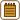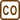## Speed of Heating Water in the Electric Kettle

### Task number: 1792

How much time will an electric kettle with a power input of 1.2 kW and efficiency of 90 % need to boil 2.0 kg of water, whose initial temperature is 10 °C?

Note: Heat loss as the result of heating the kettle itself is included in the efficiency of the kettle, therefore there is no need to take into account the heat capacity of the kettle.

• #### Notation

 P = 1.2 kW = 1.2×103 W power input of the kettle η = 90 % = 0.9 efficiency of the kettle m = 2.0 kg mass of the heated water ti = 10 °C initial water temperature τ = ? time of heating

Other necessary values

 cw = 4180 Jkg−1K−1 specific heat capacity of water tw = 100 °C boiling point of water
• #### Analysis

First of all, we need to determine the amount of heat required for the water to reach the desired temperature. This amount of energy has to be supplied by the electric kettle, whose power output we can compute from its power input and efficiency.

• #### Solution

The heat needs to be supplied to the water $$Q=c_w m (t_w - t_i)$$.

Kettle’s power is equal to $$\eta P$$, which means that during the time $$\tau$$ the kettle will provide the energy $$E= \eta P \tau$$.

The kettle has to supply the energy required to heat the water. Thus it holds:

$E=Q$

$\eta P \tau = c_w m (t_w - t_i)\,.$

From the last equation we can express the desired time of heating:

$\tau = \frac {c_w m (t_w - t_i)}{\eta P} = \frac {4180 \cdot{2} \cdot{(100-10)}}{0.9 \cdot{ 1200}} \,\mathrm s = 697 \,\mathrm s \dot= 12 \,\mathrm {min}\,.$

• #### Answer

The kettle will bring the water to the boil approximately in 12 minutes.